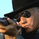‎57936‎ مشاهدة
// Script created by JoinFree
// Buy Long when you see a Green colour bar
// Sell Short when you see a Red colour bar

```study(title="GreenRedSignal", shorttitle="BuySellSignal", overlay=true)
// Script created by JoinFree
// Buy Long when you see a Green colour bar
// Sell Short when you see a Red colour bar
mysignal = ema(close, 12) - ema(close, 26)
barcolor(mysignal > mysignal ? green : red)
source = close
length = input(20, minval=1), mult = input(2.0, minval=0.001, maxval=50)
basis = sma(source, length)
dev = mult * stdev(source, length)
upper = basis + dev
lower = basis - dev
plot(basis, color=red)
p1 = plot(upper, color=blue)
p2 = plot(lower, color=blue)
fill(p1, p2)```

## التعليقاتThis is a good script. What is the actual formula? It looks like a variation of Heikin Ashi candles.
رد